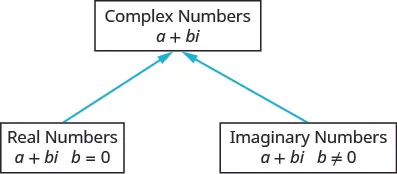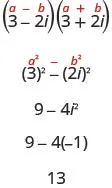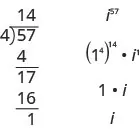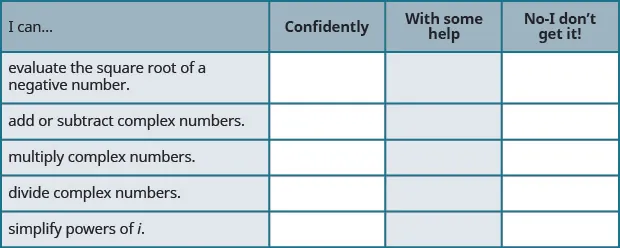Intermediate Algebra

# 8.8Use the Complex Number System

Intermediate Algebra8.8 Use the Complex Number System

### Learning Objectives

By the end of this section, you will be able to:
• Evaluate the square root of a negative number
• Add and subtract complex numbers
• Multiply complex numbers
• Divide complex numbers
• Simplify powers of $ii$

### Be Prepared 8.8

Before you get started, take this readiness quiz.

1. Given the numbers $−4,−7,0.5–,73,3,81,−4,−7,0.5–,73,3,81,$ list the rational numbers, irrational numbers, real numbers.
If you missed this problem, review Example 1.42.
2. Multiply: $(x−3)(2x+5).(x−3)(2x+5).$
If you missed this problem, review Example 5.28.
3. Rationalize the denominator:$55−3.55−3.$
If you missed this problem, review Example 5.32.

### Evaluate the Square Root of a Negative Number

Whenever we have a situation where we have a square root of a negative number we say there is no real number that equals that square root. For example, to simplify $−1,−1,$ we are looking for a real number x so that x2 = –1. Since all real numbers squared are positive numbers, there is no real number that equals –1 when squared.

Mathematicians have often expanded their numbers systems as needed. They added 0 to the counting numbers to get the whole numbers. When they needed negative balances, they added negative numbers to get the integers. When they needed the idea of parts of a whole they added fractions and got the rational numbers. Adding the irrational numbers allowed numbers like $5.5.$ All of these together gave us the real numbers and so far in your study of mathematics, that has been sufficient.

But now we will expand the real numbers to include the square roots of negative numbers. We start by defining the imaginary unit $ii$ as the number whose square is –1.

### Imaginary Unit

The imaginary unit i is the number whose square is –1.

$i2=−1ori=−1i2=−1ori=−1$

We will use the imaginary unit to simplify the square roots of negative numbers.

### Square Root of a Negative Number

If b is a positive real number, then

$−b=bi−b=bi$

We will use this definition in the next example. Be careful that it is clear that the i is not under the radical. Sometimes you will see this written as $−b=ib−b=ib$ to emphasize the i is not under the radical. But the $−b=bi−b=bi$ is considered standard form.

### Example 8.76

Write each expression in terms of i and simplify if possible:

$−25−25$ $−7−7$ $−12.−12.$

### Try It 8.151

Write each expression in terms of i and simplify if possible:

$−81−81$ $−5−5$ $−18.−18.$

### Try It 8.152

Write each expression in terms of i and simplify if possible:

$−36−36$ $−3−3$ $−27.−27.$

Now that we are familiar with the imaginary number i, we can expand the real numbers to include imaginary numbers. The complex number system includes the real numbers and the imaginary numbers. A complex number is of the form a + bi, where a, b are real numbers. We call a the real part and b the imaginary part.

### Complex Number

A complex number is of the form a + bi, where a and b are real numbers.A complex number is in standard form when written as $a+bi,a+bi,$ where a and b are real numbers.

If $b=0,b=0,$ then $a+bia+bi$ becomes $a+0·i=a,a+0·i=a,$ and is a real number.

If $b≠0,b≠0,$ then $a+bia+bi$ is an imaginary number.

If $a=0,a=0,$ then $a+bia+bi$ becomes $0+bi=bi,0+bi=bi,$ and is called a pure imaginary number.

We summarize this here.

 $a+bia+bi$ $b=0b=0$ $a+0·iaa+0·ia$ Real number $b≠0b≠0$ $a+bia+bi$ Imaginary number $a=0a=0$ $0+bibi0+bibi$ Pure imaginary number

The standard form of a complex number is $a+bi,a+bi,$ so this explains why the preferred form is $−b=bi−b=bi$ when $b>0.b>0.$

The diagram helps us visualize the complex number system. It is made up of both the real numbers and the imaginary numbers.### Add or Subtract Complex Numbers

We are now ready to perform the operations of addition, subtraction, multiplication and division on the complex numbers—just as we did with the real numbers.

Adding and subtracting complex numbers is much like adding or subtracting like terms. We add or subtract the real parts and then add or subtract the imaginary parts. Our final result should be in standard form.

### Example 8.77

Add: $−12+−27.−12+−27.$

### Try It 8.153

Add: $−8+−32.−8+−32.$

### Try It 8.154

Add: $−27+−48.−27+−48.$

Remember to add both the real parts and the imaginary parts in this next example.

### Example 8.78

Simplify: $(4−3i)+(5+6i)(4−3i)+(5+6i)$ $(2−5i)−(5−2i).(2−5i)−(5−2i).$

### Try It 8.155

Simplify: $(2+7i)+(4−2i)(2+7i)+(4−2i)$ $(8−4i)−(2−i).(8−4i)−(2−i).$

### Try It 8.156

Simplify: $(3−2i)+(−5−4i)(3−2i)+(−5−4i)$ $(4+3i)−(2−6i).(4+3i)−(2−6i).$

### Multiply Complex Numbers

Multiplying complex numbers is also much like multiplying expressions with coefficients and variables. There is only one special case we need to consider. We will look at that after we practice in the next two examples.

### Example 8.79

Multiply: $2i(7−5i).2i(7−5i).$

### Try It 8.157

Multiply: $4i(5−3i).4i(5−3i).$

### Try It 8.158

Multiply: $−3i(2+4i).−3i(2+4i).$

In the next example, we multiply the binomials using the Distributive Property or FOIL.

### Example 8.80

Multiply: $(3+2i)(4−3i).(3+2i)(4−3i).$

### Try It 8.159

Multiply: $(5−3i)(−1−2i).(5−3i)(−1−2i).$

### Try It 8.160

Multiply: $(−4−3i)(2+i).(−4−3i)(2+i).$

In the next example, we could use FOIL or the Product of Binomial Squares Pattern.

### Example 8.81

Multiply: $(3+2i)2(3+2i)2$

### Try It 8.161

Multiply using the Binomial Squares pattern: $(−2−5i)2.(−2−5i)2.$

### Try It 8.162

Multiply using the Binomial Squares pattern: $(−5+4i)2.(−5+4i)2.$

Since the square root of a negative number is not a real number, we cannot use the Product Property for Radicals. In order to multiply square roots of negative numbers we should first write them as complex numbers, using $−b=bi.−b=bi.$ This is one place students tend to make errors, so be careful when you see multiplying with a negative square root.

### Example 8.82

Multiply: $−36·−4.−36·−4.$

### Try It 8.163

Multiply: $−49·−4.−49·−4.$

### Try It 8.164

Multiply: $−36·−81.−36·−81.$

In the next example, each binomial has a square root of a negative number. Before multiplying, each square root of a negative number must be written as a complex number.

### Example 8.83

Multiply: $(3−−12)(5+−27).(3−−12)(5+−27).$

### Try It 8.165

Multiply: $(4−−12)(3−−48).(4−−12)(3−−48).$

### Try It 8.166

Multiply: $(−2+−8)(3−−18).(−2+−8)(3−−18).$

We first looked at conjugate pairs when we studied polynomials. We said that a pair of binomials that each have the same first term and the same last term, but one is a sum and one is a difference is called a conjugate pair and is of the form $(a−b),(a+b).(a−b),(a+b).$

A complex conjugate pair is very similar. For a complex number of the form $a+bi,a+bi,$ its conjugate is $a−bi.a−bi.$ Notice they have the same first term and the same last term, but one is a sum and one is a difference.

### Complex Conjugate Pair

A complex conjugate pair is of the form $a+bi,a+bi,$$a−bi.a−bi.$

We will multiply a complex conjugate pair in the next example.

### Example 8.84

Multiply: $(3−2i)(3+2i).(3−2i)(3+2i).$

### Try It 8.167

Multiply: $(4−3i)·(4+3i).(4−3i)·(4+3i).$

### Try It 8.168

Multiply: $(−2+5i)·(−2−5i).(−2+5i)·(−2−5i).$

From our study of polynomials, we know the product of conjugates is always of the form $(a−b)(a+b)=a2−b2.(a−b)(a+b)=a2−b2.$ The result is called a difference of squares. We can multiply a complex conjugate pair using this pattern.

The last example we used FOIL. Now we will use the Product of Conjugates Pattern.Notice this is the same result we found in Example 8.84.

When we multiply complex conjugates, the product of the last terms will always have an $i2i2$ which simplifies to $−1.−1.$

$(a−bi)(a+bi)a2−(bi)2a2−b2i2a2−b2(−1)a2+b2(a−bi)(a+bi)a2−(bi)2a2−b2i2a2−b2(−1)a2+b2$

This leads us to the Product of Complex Conjugates Pattern: $(a−bi)(a+bi)=a2+b2(a−bi)(a+bi)=a2+b2$

### Product of Complex Conjugates

If a and b are real numbers, then

$(a−bi)(a+bi)=a2+b2(a−bi)(a+bi)=a2+b2$

### Example 8.85

Multiply using the Product of Complex Conjugates Pattern: $(8−2i)(8+2i).(8−2i)(8+2i).$

### Try It 8.169

Multiply using the Product of Complex Conjugates Pattern: $(3−10i)(3+10i).(3−10i)(3+10i).$

### Try It 8.170

Multiply using the Product of Complex Conjugates Pattern: $(−5+4i)(−5−4i).(−5+4i)(−5−4i).$

### Divide Complex Numbers

Dividing complex numbers is much like rationalizing a denominator. We want our result to be in standard form with no imaginary numbers in the denominator.

### Example 8.86

#### How to Divide Complex Numbers

Divide: $4+3i3−4i.4+3i3−4i.$

### Try It 8.171

Divide: $2+5i5−2i.2+5i5−2i.$

### Try It 8.172

Divide: $1+6i6−i.1+6i6−i.$

We summarize the steps here.

### How To

#### How to divide complex numbers.

1. Step 1. Write both the numerator and denominator in standard form.
2. Step 2. Multiply the numerator and denominator by the complex conjugate of the denominator.
3. Step 3. Simplify and write the result in standard form.

### Example 8.87

Divide, writing the answer in standard form: $−35+2i.−35+2i.$

### Try It 8.173

Divide, writing the answer in standard form: $41−4i.41−4i.$

### Try It 8.174

Divide, writing the answer in standard form: $−2−1+2i.−2−1+2i.$

Be careful as you find the conjugate of the denominator.

### Example 8.88

Divide: $5+3i4i.5+3i4i.$

### Try It 8.175

Divide: $3+3i2i.3+3i2i.$

### Try It 8.176

Divide: $2+4i5i.2+4i5i.$

### Simplify Powers of i

The powers of $ii$ make an interesting pattern that will help us simplify higher powers of i. Let’s evaluate the powers of $ii$ to see the pattern.

$i1i2i3i4i−1i2·ii2·i2−1·i(−1)(−1) −i1 i5i6i7i8 i4·ii4·i2i4·i3i4·i4 1·i1·i21·i31·1 ii2i31 −1−ii1i2i3i4i−1i2·ii2·i2−1·i(−1)(−1) −i1 i5i6i7i8 i4·ii4·i2i4·i3i4·i4 1·i1·i21·i31·1 ii2i31 −1−i$

We summarize this now.

$i1=ii5=i i2=−1i6=−1 i3=−ii7=−i i4=1i8=1i1=ii5=i i2=−1i6=−1 i3=−ii7=−i i4=1i8=1$

If we continued, the pattern would keep repeating in blocks of four. We can use this pattern to help us simplify powers of i. Since i4 = 1, we rewrite each power, in, as a product using i4 to a power and another power of i.

We rewrite it in the form $in=(i4)q·ir,in=(i4)q·ir,$ where the exponent, q, is the quotient of n divided by 4 and the exponent, r, is the remainder from this division. For example, to simplify i57, we divide 57 by 4 and we get 14 with a remainder of 1. In other words, $57=4·14+1.57=4·14+1.$ So we write $i57=(14)14·i1i57=(14)14·i1$ and then simplify from there.### Example 8.89

Simplify: $i86.i86.$

### Try It 8.177

Simplify: $i75.i75.$

### Try It 8.178

Simplify: $i92.i92.$

### Media

Access these online resources for additional instruction and practice with the complex number system.

### Section 8.8 Exercises

#### Practice Makes Perfect

Evaluate the Square Root of a Negative Number

In the following exercises, write each expression in terms of i and simplify if possible.

409.

$−16−16$ $−11−11$
$−8−8$

410.

$−121−121$ $−1−1$ $−20−20$

411.

$−100−100$ $−13−13$ $−45−45$

412.

$−49−49$ $−15−15$ $−75−75$

Add or Subtract Complex Numbers In the following exercises, add or subtract.

413.

$−75 + −48 −75 + −48$

414.

$−12 + −75 −12 + −75$

415.

$−50 + −18 −50 + −18$

416.

$−72 + −8 −72 + −8$

417.

$( 1 + 3 i ) + ( 7 + 4 i ) ( 1 + 3 i ) + ( 7 + 4 i )$

418.

$( 6 + 2 i ) + ( 3 − 4 i ) ( 6 + 2 i ) + ( 3 − 4 i )$

419.

$( 8 − i ) + ( 6 + 3 i ) ( 8 − i ) + ( 6 + 3 i )$

420.

$( 7 − 4 i ) + ( −2 − 6 i ) ( 7 − 4 i ) + ( −2 − 6 i )$

421.

$( 1 − 4 i ) − ( 3 − 6 i ) ( 1 − 4 i ) − ( 3 − 6 i )$

422.

$( 8 − 4 i ) − ( 3 + 7 i ) ( 8 − 4 i ) − ( 3 + 7 i )$

423.

$( 6 + i ) − ( −2 − 4 i ) ( 6 + i ) − ( −2 − 4 i )$

424.

$( −2 + 5 i ) − ( −5 + 6 i ) ( −2 + 5 i ) − ( −5 + 6 i )$

425.

$( 5 − −36 ) + ( 2 − −49 ) ( 5 − −36 ) + ( 2 − −49 )$

426.

$( −3 + −64 ) + ( 5 − −16 ) ( −3 + −64 ) + ( 5 − −16 )$

427.

$( −7 − −50 ) − ( −32 − −18 ) ( −7 − −50 ) − ( −32 − −18 )$

428.

$( −5 + −27 ) − ( −4 − −48 ) ( −5 + −27 ) − ( −4 − −48 )$

Multiply Complex Numbers

In the following exercises, multiply.

429.

$4 i ( 5 − 3 i ) 4 i ( 5 − 3 i )$

430.

$2 i ( −3 + 4 i ) 2 i ( −3 + 4 i )$

431.

$−6 i ( −3 − 2 i ) −6 i ( −3 − 2 i )$

432.

$− i ( 6 + 5 i ) − i ( 6 + 5 i )$

433.

$( 4 + 3 i ) ( −5 + 6 i ) ( 4 + 3 i ) ( −5 + 6 i )$

434.

$( −2 − 5 i ) ( −4 + 3 i ) ( −2 − 5 i ) ( −4 + 3 i )$

435.

$( −3 + 3 i ) ( −2 − 7 i ) ( −3 + 3 i ) ( −2 − 7 i )$

436.

$( −6 − 2 i ) ( −3 − 5 i ) ( −6 − 2 i ) ( −3 − 5 i )$

In the following exercises, multiply using the Product of Binomial Squares Pattern.

437.

$( 3 + 4 i ) 2 ( 3 + 4 i ) 2$

438.

$( −1 + 5 i ) 2 ( −1 + 5 i ) 2$

439.

$( −2 − 3 i ) 2 ( −2 − 3 i ) 2$

440.

$( −6 − 5 i ) 2 ( −6 − 5 i ) 2$

In the following exercises, multiply.

441.

$−25 · −36 −25 · −36$

442.

$−4 · −16 −4 · −16$

443.

$−9 · −100 −9 · −100$

444.

$−64 · −9 −64 · −9$

445.

$( −2 − −27 ) ( 4 − −48 ) ( −2 − −27 ) ( 4 − −48 )$

446.

$( 5 − −12 ) ( −3 + −75 ) ( 5 − −12 ) ( −3 + −75 )$

447.

$( 2 + −8 ) ( −4 + −18 ) ( 2 + −8 ) ( −4 + −18 )$

448.

$( 5 + −18 ) ( −2 − −50 ) ( 5 + −18 ) ( −2 − −50 )$

449.

$( 2 − i ) ( 2 + i ) ( 2 − i ) ( 2 + i )$

450.

$( 4 − 5 i ) ( 4 + 5 i ) ( 4 − 5 i ) ( 4 + 5 i )$

451.

$( 7 − 2 i ) ( 7 + 2 i ) ( 7 − 2 i ) ( 7 + 2 i )$

452.

$( −3 − 8 i ) ( −3 + 8 i ) ( −3 − 8 i ) ( −3 + 8 i )$

In the following exercises, multiply using the Product of Complex Conjugates Pattern.

453.

$( 7 − i ) ( 7 + i ) ( 7 − i ) ( 7 + i )$

454.

$( 6 − 5 i ) ( 6 + 5 i ) ( 6 − 5 i ) ( 6 + 5 i )$

455.

$( 9 − 2 i ) ( 9 + 2 i ) ( 9 − 2 i ) ( 9 + 2 i )$

456.

$( −3 − 4 i ) ( −3 + 4 i ) ( −3 − 4 i ) ( −3 + 4 i )$

Divide Complex Numbers

In the following exercises, divide.

457.

$3 + 4 i 4 − 3 i 3 + 4 i 4 − 3 i$

458.

$5 − 2 i 2 + 5 i 5 − 2 i 2 + 5 i$

459.

$2 + i 3 − 4 i 2 + i 3 − 4 i$

460.

$3 − 2 i 6 + i 3 − 2 i 6 + i$

461.

$3 2 − 3 i 3 2 − 3 i$

462.

$2 4 − 5 i 2 4 − 5 i$

463.

$−4 3 − 2 i −4 3 − 2 i$

464.

$−1 3 + 2 i −1 3 + 2 i$

465.

$1 + 4 i 3 i 1 + 4 i 3 i$

466.

$4 + 3 i 7 i 4 + 3 i 7 i$

467.

$−2 − 3 i 4 i −2 − 3 i 4 i$

468.

$−3 − 5 i 2 i −3 − 5 i 2 i$

Simplify Powers of i

In the following exercises, simplify.

469.

$i 41 i 41$

470.

$i 39 i 39$

471.

$i 66 i 66$

472.

$i 48 i 48$

473.

$i 128 i 128$

474.

$i 162 i 162$

475.

$i 137 i 137$

476.

$i 255 i 255$

#### Writing Exercises

477.

Explain the relationship between real numbers and complex numbers.

478.

Aniket multiplied as follows and he got the wrong answer. What is wrong with his reasoning?

$−7 · −7 49 7 −7 · −7 49 7$

479.

Why is $−64=8i−64=8i$ but $−643=−4.−643=−4.$

480.

Explain how dividing complex numbers is similar to rationalizing a denominator.

#### Self Check

After completing the exercises, use this checklist to evaluate your mastery of the objectives of this section.On a scale of $1−10,1−10,$ how would you rate your mastery of this section in light of your responses on the checklist? How can you improve this?

Order a print copy

As an Amazon Associate we earn from qualifying purchases.